JalFaizy Shaikh — Published On December 5, 2016 and Last Modified On July 5th, 2020

## Introduction

Tree Based algorithms like Random Forest, Decision Tree, and Gradient Boosting are commonly used machine learning algorithms. Tree based algorithms are often used to solve data science problems. Every data science aspirant must be skilled in tree based algorithms. We conducted this skill test to help you analyze your knowledge in these algorithms.

A total of 1016 participants registered for this skill test. The test was designed to test the conceptual knowledge of tree based algorithms. If you are one of those who missed out on this skill test, here are the questions and solutions. You missed on the real time test, but can read this article to find out how you could have answered correctly.

Here are the leaderboard ranking for all the participants.## Overall ResultsYou can access the final scores here. More than 400 people participated in the skill test and the highest score obtained was 36 . Here are a few statistics about the distribution.

Mean Score : 16.98

Median Score : 19

Mode Score : 19

You can see that got a bi-modal distribution of scores. We were not expecting that as the first 8 questions were relatively easy and could be solved grounds up without too much knowledge about decision trees.

If you did well, here is another test coming up – Skill test – Regression , which would test you on knowledge of solving regression problems.

## Helpful Resources on Tree Based Algorithms

Here are a few resources you can refer to to improve your knowledge on tree based algorithms.

A Complete Tutorial on Tree Based Modeling from Scratch (in R & Python)

Introduction to Random forest – Simplified

Complete Guide to Parameter Tuning in Gradient Boosting (GBM) in Python

## Questions and Solutions

Q 1) The data scientists at “BigMart Inc” have collected 2013 sales data for 1559 products across 10 stores in different cities. Also, certain attributes of each product based on these attributes and store have been defined. The aim is to build a predictive model and find out the sales of each product at a particular store during a defined period.

Which learning problem does this belong to?

1. Supervised learning
2. Unsupervised learning
3. Reinforcement learning
4. None

Solution: A

Supervised learning is the machine learning task of inferring a function from labeled training data. Here historical sales data is our training data and it contains the labels / outcomes.

Q2) Before building our model, we first look at our data and make predictions manually. Suppose we have only one feature as an independent variable (Outlet_Location_Type) along with a continuous dependent variable (Item_Outlet_Sales).

 Outlet_Location_Type Item_Outlet_Sales Tier 1 3735.14 Tier 3 443.42 Tier 1 2097.27 Tier 3 732.38 Tier 3 994.71

We see that we can possibly differentiate in Sales based on location (tier 1 or tier 3). We can write simple if-else statements to make predictions.

Which of the following models could be used to generate predictions (may not be most accurate)?

1. if “Outlet_Location” is “Tier 1”: then “Outlet_Sales” is 2000, else “Outlet_Sales” is 1000
2. if “Outlet_Location” is “Tier 1”: then “Outlet_Sales” is 1000, else “Outlet_Sales” is 2000
3. if “Outlet_Location” is “Tier 3”: then “Outlet_Sales” is 500, else “Outlet_Sales” is 5000
4. Any of the above

Solution: D

All the options would be correct. All the above models give a prediction as output and here we are not talking about most or least accurate.

Q3) The below created if-else statement is called a decision stump:

Our model: if “Outlet_Location” is “Tier 1”: then “Outlet_Sales” is 2000, else “Outlet_Sales” is 1000

Now let us evaluate the model we created above on following data:

Evaluation Data:

 Outlet_Location_Type Item_Outlet_Sales Tier 1 3735.1380 Tier 3 443.4228 Tier 1 2097.2700 Tier 3 732.3800 Tier 3 994.7052

We will calculate RMSE to evaluate this model.

The root-mean-square error (RMSE) is a measure of the differences between values predicted by a model or an estimator and the values actually observed.

The formula is :

`rmse = (sqrt(sum(square(predicted_values - actual_values)) / number of observations))`

What would be the RMSE value for this model?

1. ~23
2. ~824
3. ~680318
4. ~2152

Solution: B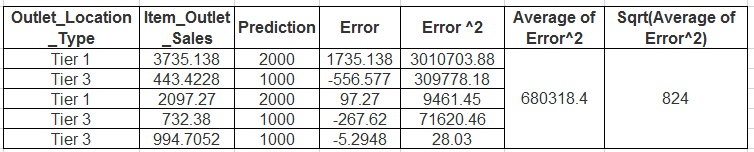So by calculating RMSE value using the formula above, we get ~824 as our answer.

Q4) For the same data, let us evaluate our models. The root-mean-square error (RMSE) is a measure of the differences between values predicted by a model or an estimator and the values actually observed.

 Outlet_Location_Type Item_Outlet_Sales Tier 1 3735.1380 Tier 3 443.4228 Tier 1 2097.2700 Tier 3 732.3800 Tier 3 994.7052

The formula is :

`rmse = (sqrt(sum(square(predicted_values - actual_values)) / num_samples))`

Which of the following will be the best model with respect to RMSE scoring?

1. if “Outlet_Location_Type” is “Tier 1”: then “Outlet_Sales” is 2000, else “Outlet_Sales” is 1000
2. if “Outlet_Location_Type” is “Tier 1”: then “Outlet_Sales” is 1000, else “Outlet_Sales” is 2000
3. if “Outlet_Location_Type” is “Tier 3”: then “Outlet_Sales” is 500, else “Outlet_Sales” is 5000
4. if “Outlet_Location_Type” is “Tier 3”: then “Outlet_Sales” is 2000, else “Outlet_Sales” is 200

Solution: A

Calculate the RMSE value for each if-else model:

1. RMSE value of the model: 824.81
2. RMSE value of the model: 1656.82
3. RMSE value of the model: 1437.19
4. RMSE value of the model: 2056.07

We see that the model in option A has the lowest value and lower the RMSE, better the model.

Q5) Now let’s take multiple features into account.

 Outlet_Location_Type Outlet_Type Item_Outlet_Sales Tier 1 Supermarket Type1 3735.1380 Tier 3 Supermarket Type2 443.4228 Tier 1 Supermarket Type1 2097.2700 Tier 3 Grocery Store 732.3800 Tier 3 Supermarket Type1 994.7052

If have multiple if-else ladders, which model is best with respect to RMSE?

1. ```if “Outlet_Location_Type” is 'Tier 1':
return 2500
else:
if “Outlet_Type” is 'Supermarket Type1':
return 1000
elif “Outlet_Type” is 'Supermarket Type2':
return 400
else:
return 700```
2. ```if "Outlet_Location_Type" is 'Tier 3':
return 2500
else:
if "Outlet_Type" is 'Supermarket Type1':
return 1000
elif "Outlet_Type" is 'Supermarket Type2':
return 400
else:
return 700```
3. ```if "Outlet_Location_Type" is 'Tier 3':
return 3000
else:
if "Outlet_Type" is 'Supermarket Type1':
return 1000
else:
return 500```
4. ```if "Outlet_Location_Type" is 'Tier 1':
return 3000
else:
if "Outlet_Type" is 'Supermarket Type1':
return 1000
else:
return 450```

Solution: D

1. RMSE value: 581.50
2. RMSE value: 1913.36
3. RMSE value: 2208.36
4. RMSE value: 535.75

We see that option D has the lowest value

Q6) Till now, we have just created predictions using some intuition based rules. Hence our predictions may not be optimal.What could be done to optimize the approach of finding better predictions from the given data?

1. Put predictions which are the sum of all the actual values of samples present. For example, in “Tier 1”, we have two values 3735.1380 and 2097.2700, so we will take ~5832 as our prediction
2. Put predictions which are the difference of all the actual values of samples present. For example, in “Tier 1”, we have two values 3735.1380 and 2097.2700, so we will take ~1638 as our prediction
3. Put predictions which are mean of all the actual values of samples present. For example, in “Tier 1”, we have two values 3735.1380 and 2097.2700, so we will take ~2916 as our prediction

Solution: C

We will take that value which is more representative of the data. Given all three options, central tendency, mean value would be a better fit for the data.

Q7) We could improve our model by selecting the feature which gives a better prediction when we use it for splitting (It is a process of dividing a node into two or more sub-nodes).

 Outlet_Location_Type Item_Fat_Content Item_Outlet_Sales Tier 1 Low Fat 3735.1380 Tier 3 Regular 443.4228 Tier 1 Low Fat 2097.2700 Tier 3 Regular 732.3800 Tier 3 Low Fat 994.7052

In this example, we want to find which feature would be better for splitting root node (entire population or sample and this further gets divided into two or more homogeneous sets).

Assume splitting method is “Reduction in Variance” i.e. we split using a variable, which results in overall lower variance.What is the resulting variance if we split using Outlet_Location_Type?

1. ~298676
2. ~298676
3. ~3182902
4. ~2222733
5. None of these

Solution: A

Option A is correct. The steps to solve this problem are:

• Calculate mean of target value for “Tier 1” and then find the variance of each of the target values of “Tier 1”
• Similarly calculate the variance for “Tier 3”
• Find weighted mean of variance of “Tier 1” and “Tier 3” (above calculated values).

P.S. You will need to take weigthed mean.

Q8) Next, we want to find which feature would be better for splitting root node (where root node represents entire population). For this, we will set “Reduction in Variance” as our splitting method.

 Outlet_Location_Type Item_Fat_Content Item_Outlet_Sales Tier 1 Low Fat 3735.1380 Tier 3 Regular 443.4228 Tier 1 Low Fat 2097.2700 Tier 3 Regular 732.3800 Tier 3 Low Fat 994.7052

The split with lower variance is selected as the criteria to split the population.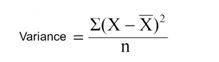Among Between Outlet_Location_Type and Item_Fat_Content, which was a better feature to split?

1. Outlet_Location_Type
2. Item_Fat_Content
3. will not split on both

Solution: A

Option A is correct because Outlet_Location_Type has more reduction in variance. You can perform calculation similar to last question.

Q9) Look at the below image: The red dots represent original data input, while the green line is the resultant model.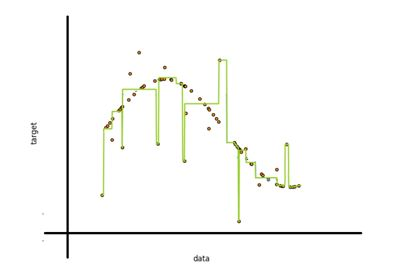How do you propose to make this model better while working with decision tree?

1. Let it be. The model is general enough
2. Set the number of nodes in the tree beforehand so that it does not overdo its task
3. Build a decision tree model, use cross validation method to tune tree parameters
4. Both B and C
5. All A, B and C
6. None of these

Solution: C

A. As we can see in the image, our model is not general enough, it takes outliers/ noise into account when calculating predictions which makes it overfit the data.

B. If we can set the number of nodes, we could easily get an optimal tree. But to select this value optimally beforehand is very hard, as it requires extensive cross-validation to be generalizable.

C. Tuning Tree parameters is the best method to ensure generalizability

Q10) Which methodology does Decision Tree (ID3) take to decide on first split?

1. Greedy approach
3. Brute force approach
4. None of these

Solution: A

The process of top-down induction of decision trees (TDIDT) is an example of a greedy algorithm, and it is by far the most common strategy for learning decision trees from data. Read here.

Q11) There are 24 predictors in a dataset. You build 2 models on the dataset:

1. Bagged decision trees and
2. Random forest

Let the number of predictors used at a single split in bagged decision tree is A and Random Forest is B.

Which of the following statement is correct?

1. A >= B
2. A < B
3. A >> B
4. Cannot be said since different iterations use different numbers of predictors

Solution: A

Random Forest uses a subset of predictors for model building, whereas bagged trees use all the features at once.

Q12) Why do we prefer information gain over accuracy when splitting?

1. Decision Tree is prone to overfit and accuracy doesn’t help to generalize
2. Information gain is more stable as compared to accuracy
3. Information gain chooses more impactful features closer to root
4. All of these

Solution: D

All the above options are correct

Q13) Random forests (While solving a regression problem) have the higher variance of predicted result in comparison to Boosted Trees (Assumption: both Random Forest and Boosted Tree are fully optimized).

1. True
2. False
3. Cannot be determined

Solution: C

It completely depends on the data, the assumption cannot be made without data.

Q14) Assume everything else remains same, which of the following is the right statement about the predictions from decision tree in comparison with predictions from Random Forest?

1. Lower Variance, Lower Bias
2. Lower Variance, Higher Bias
3. Higher Variance, Higher Bias
4. Lower Bias, Higher Variance

Solution: D

The predicted values in Decision Trees have low Bias but high Variance when compared to Random Forests. This is because random forest attempts to reduce variance by bootstrap aggregation. Refer topic 15.4 of Elements of Statistical Learning

Q15) Which of the following tree based algorithm uses some parallel (full or partial) implementation?

1. Random Forest
3. XGBOOST
4. Both A and C
5. A, B and C

Solution: D

Only Random Forest and XGBoost have parallel implementations.

Random Forest is very easy to parallelize, where as XGBoost can have partially parallel implementation. In Random Forest, all trees grows parallel and finally ensemble the output of each tree .

Xgboost doesn’t run multiple trees in parallel like Random Forest, you need predictions after each tree to update gradients. Rather it does the parallelization WITHIN a single tree to create branches independently.

Q16) Which of the following could not be result of two-dimensional feature space from natural recursive binary split?1. 1 only
2. 2 only
3. 1 and 2
4. None

Solution: A

1 is not possible. Therefore, Option A is correct. For more details, refer to Page 308 from ELSI (Elements of Statistical Learning).

Q17) Which of the following is not possible in a boosting algorithm?

1. Increase in training error.
2. Decrease in training error
3. Increase in testing error
4. Decrease in testing error
5. Any of the above

Solution: A

Boosted algorithms minimize error in previously predicted values by last estimator. So it always decreases training error.

Q18) Which of the following is a decision boundary of Decision Tree?1. B
2. A
3. D
4. C
5. Can’t Say

Solution: C

Decision Boundaries of decision trees are always perpendicular to X and Y axis.

Q19) Let’s say we have m numbers of estimators (trees) in a boosted tree. Now, how many intermediate trees will work on modified version (OR weighted) of data set?

1. 1
2. m-1
3. m
4. Can’t say
5. None of the above

Solution: B

The first tree in boosted trees works on the original data, whereas all the rest work on modified version of the data.

Q20) Boosted decision trees perform better than Logistic Regression on anomaly detection problems (Imbalanced Class problems).

1. True, because they give more weight for lesser weighted class in successive rounds
2. False, because boosted trees are based on Decision Tree, which will try to overfit the data

Solution: A

Option A is correct

Q21) Provided n < N and m < M. A Bagged Decision Tree with a dataset of N rows and M columns uses____rows and ____ columns for training an individual intermediate tree.

1. N, M
2. N, M
3. n, M
4. n, m

Solution: C

Bagged trees uses all the columns for only a sample of the rows. So randomization is done on the number of observations not on number of columns.

Q22) Given 1000 observations, Minimum observation required to split a node equals to 200 and minimum leaf size equals to 300 then what could be the maximum depth of a decision tree?

1. 1
2. 2
3. 3
4. 4
5. 5

Solution: B

The leaf nodes will be as follows for minimum observation to split is 200 and minimum leaf size is 300: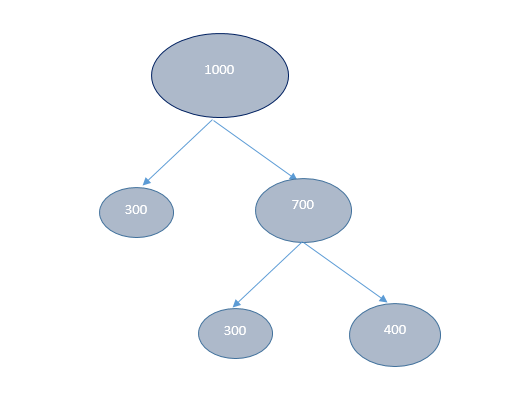So only after 2 split, the tree is created. Therefore depth is 2.

Q23) Consider a classification tree for whether a person watches ‘Game of Thrones’ based on features like age, gender, qualification and salary. Is it possible to have following leaf node?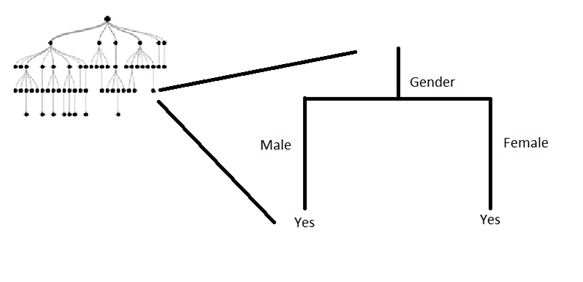1. Yes
2. No
3. Can’t say

Solution: A

A node can be split on a feature, as long as it gives information after split. So even though the above split does not reduce the classification error, it improves the Gini index and the cross-entropy. Refer Pg. 314 of ISLR.

Q24) Generally, in terms of prediction performance which of the following arrangements are correct:

1. Bagging>Boosting>Random Forest>Single Tree
2. Boosting>Random Forest>Single Tree>Bagging
3. Boosting>Random Forest>Bagging>Single Tree
4. Boosting >Bagging>Random Forest>Single Tree

Solution: C

Generally speaking, Boosting algorithms will perform better than bagging algorithms. In terms of bagging vs random forest, random forest works better in practice because random forest has less correlated trees compared to bagging. And it’s always true that ensembles of algorithms are better than single models

Q25) In which of the following application(s), a tree based algorithm can be applied successfully?

1. Recognizing moving hand gestures in real time
2. Predicting next move in a chess game
3. Predicting sales values of a company based on their past sales
4. A and B
5. A, B, and C

Solution: E

Option E is correct as we can apply tree based algorithm in all the 3 scenarios.

Q26) When using Random Forest for feature selection, suppose you permute values of two features – A and B. Permutation is such that you change the indices of individual values so that they do not remain associated with the same target as before.

For example: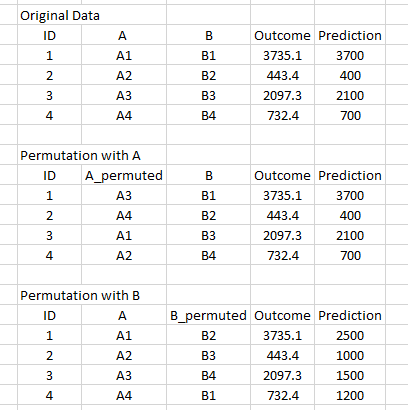You notice that permuting values does not affect the score of model built on A, whereas the score decreases on the model trained on B.Which of the following features would you select from the following solely based on the above finding?

1. (A)
2. (B)

Solution: B

This is called mean decrease in accuracy when using random forest for feature selection. Intuitively, if shuffling the values is not impacting the predictions, the feature is unlikely to add value.

Q27) Boosting is said to be a good classifier because:

1. It creates all ensemble members in parallel, so their diversity can be boosted.
2. It attempts to minimize the margin distribution
3. It attempts to maximize the margins on the training data
4. None of these

Solution: B

A. Trees are sequential in boosting. They are not parallel

B. Boosting attempts to minimize residual error which reduces margin distribution

C. As we saw in B, margins are minimized and not maximized.

Therefore B is true

Q28) Which splitting algorithm is better with categorical variable having high cardinality?

1. Information Gain
2. Gain Ratio
3. Change in Variance
4. None of these

Solution: B

When high cardinality problems, gain ratio is preferred over any other splitting technique. Refer slide number 72 of this presentation.

Q29) There are “A” features in a dataset and a Random Forest model is built over it. It is given that there exists only one significant feature of the outcome – “Feature1”. What would be the % of total splits that will not consider the “Feature1” as one of the features involved in that split (It is given that m is the number of maximum features for random forest)?

Note: Considering random forest select features space for every node split.

1. (A-m)/A
2. (m-A)/m
3. m/A
4. Cannot be determined

Solution: A

Option A is correct. This can be considered as permutation of not selecting a predictor from all the possible predictors

Q30) Suppose we have missing values in our data. Which of the following method(s) can help us to deal with missing values while building a decision tree?

1. Let it be. Decision Trees are not affected by missing values
2. Fill dummy value in place of missing, such as -1
3. Impute missing value with mean/median
4. All of these

Solution: D

Q31) To reduce under fitting of a Random Forest model, which of the following method can be used?

1. Increase minimum sample leaf value
2. increase depth of trees
3. Increase the value of minimum samples to split
4. None of these

Solution: B

Only option B is correct, because

A: increasing the number of samples for a leaf will reduce the depth of a tree, indirectly increasing underfitting

B: Increasing depth will definitely decrease help reduce underfitting

C: increasing the number of samples considered to split will have no effect, as the same information will be given to the model.

Therefore B is True.

Q32) While creating a Decision Tree, can we reuse a feature to split a node?

1. Yes
2. No

Solution: A

Yes, decision tree recursively uses all the features at each node.

Q33) Which of the following is a mandatory data pre-processing step(s) for XGBOOST?

1. Impute Missing Values
2. Remove Outliers
3. Convert data to numeric array / sparse matrix
4. Input variable must have normal distribution
5. Select the sample of records for each tree/ estimators
1. 1 and 2
2. 1, 2 and 3
3. 3, 4 and 5
4. 3
5. 5
6. All

Solution: D

XGBoost is doesn’t require most of the pre-processing steps, so only converting data to numeric is required among of the above listed steps

Q34) Decision Trees are not affected by multicollinearity in features:

1. TRUE
2. FALSE

Solution: A

The statement is true. For example, if there are two 90% correlated features, decision tree would consider only one of them for splitting.

Q35) For parameter tuning in a boosting algorithm, which of the following search strategies may give best tuned model:

1. Random Search.
2. Grid Search.
3. A or B
4. Can’t say

Solution: C

For a a given search space,

• Random search randomly picks out hyperparameters. In terms of time required, random search requires much less time to converge.
• Grid search deterministically tries to find optimum hyperparameters. This is a brute force approach for solving a problem, and requires much time to give output.

Both random search or grid search may give best tuned model. It depends on how much time and resources can be allocated for search.

Q36) Imagine a two variable predictor space having 10 data points. A decision tree is built over it with 5 leaf nodes. The number of distinct regions that will be formed in predictors space?

1. 25
2. 10
3. 2
4. 5

Solution: D

The predictor space will be divided into 5 regions. Therefore, option D is correct.

Q37) In Random Forest, which of the following is randomly selected?

1. Number of decision trees
2. features to be taken into account when building a tree
3. samples to be given to train individual tree in a forest
4. B and C
5. A, B and C

Solution: D

Option A is False because, number of trees has to decided when building a tree. It is not random.

Options B and C are true

Q38) Which of the following are the disadvantage of Decision Tree algorithm?

1. Decision tree is not easy to interpret
2. Decision tree is not a very stable algorithm
3. Decision Tree will over fit the data easily if it perfectly memorizes it
4. Both B and C

Solution: D

Option A is False, as decision tree are very easy to interpret

Option B is True, as decision tree are high unstable models

Option C is True, as decision tree also tries to memorize noise.

So option D is True.

Q39) While tuning the parameters “Number of estimators” and “Shrinkage Parameter”/”Learning Rate” for boosting algorithm.Which of the following relationship should be kept in mind?

1. Number of estimators is directly proportional to shrinkage parameter
2. Number of estimators is inversely proportional to shrinkage parameter
3. Both have polynomial relationship

Solution: B

It is generally seen that smaller learning rates require more trees to be added to the model and vice versa. So when tuning parameters of boosting algorithm, there is a trade-off between learning rate and number of estimators

Q40) Let’s say we have m number of estimators (trees) in a XGBOOST model. Now, how many trees will work on bootstrapped data set?

1. 1
2. m-1
3. m
4. Can’t say
5. None of the above

Solution: C

All the trees in XGBoost will work on bootstrapped data. Therefore, option C is true

Q41) Which of the following statement is correct about XGBOOST parameters:

1. Learning rate can go upto 10
2. Sub Sampling / Row Sampling percentage should lie between 0 to 1
3. Number of trees / estimators can be 1
4. Max depth can not be greater than 10
1. 1
2. 1 and 3
3. 1, 3 and 4
4. 2 and 3
5. 2
6. 4

Solution: D

1 and 4 are wrong statements, whereas 2 and 3 are correct. Therefore D is true. Refer this article.

Q42) What can be the maximum depth of decision tree (where k is the number of features and N is the number of samples)? Our constraint is that we are considering a binary decision tree with no duplicate rows in sample (Splitting criterion is not fixed).

1. N
2. N – k – 1
3. N – 1
4. k – 1

Solution: C

The answer is N-1. An example of max depth would be when splitting only happens on the left node.

Q43) Boosting is a general approach that can be applied to many statistical learning methods for regression or classification.

1. True
2. False

Solution: A

Boosting is an ensemble technique and can be applied to various base algorithms

Q44) Predictions of individual trees of bagged decision trees have lower correlation in comparison to individual trees of random forest.

1. TRUE
2. FALSE

Solution: B

This is False because random Forest has more randomly generated uncorrelated trees than bagged decision trees. Random Forest considers only a subset of total features. So individual trees that are generated by random forest may have different feature subsets. This is not true for bagged trees.

Q45) Below is a list of parameters of Decision Tree. In which of the following cases higher is better?

1. Number of samples used for split
2. Depth of tree
3. Samples for leaf
4. Can’t Say

Solution: D

For all three options A, B and C, it is not necessary that if you increase the value of parameter the performance may increase. For example, if we have a very high value of depth of tree, the resulting tree may overfit the data, and would not generalize well. On the other hand, if we have a very low value, the tree may underfit the data. So, we can’t say for sure that “higher is better”.

## End Notes

I hope you enjoyed taking the test and you found the solutions helpful. The test focused on conceptual knowledge of tree based algorithms.

We tried to clear all your doubts through this article but if we have missed out on something then let me know in comments below. If you have any suggestions or improvements you think we should make in the next skilltest, let us know in the comments below.

Don’t forget to register for Skilltest Regression coming up on 17 Dec’16. You will be tested on regression and its various forms. All the Best!

### You can test your skills and knowledge. Check out Live Competitions and compete with best Data Scientists from all over the world.###### JalFaizy Shaikh

Faizan is a Data Science enthusiast and a Deep learning rookie. A recent Comp. Sc. undergrad, he aims to utilize his skills to push the boundaries of AI research.

## 20 thoughts on "45 questions to test Data Scientists on Tree Based Algorithms (Decision tree, Random Forests, XGBoost)"###### DR Venugopala Rao Manneni says:December 05, 2016 at 7:50 am###### Utkarsh Dubey says:December 05, 2016 at 7:53 am
By bimodal distribution of score, we can infer that there are 2 types of participants: 1. Actually want to participate and completed the test 2. Entered the test casually and lost interest after few question. OR, false participants just to increase total number BTW. The test was awesome looking forward for more such skill-test even after regression one. Reply###### Faizan Shaikh says:December 05, 2016 at 8:52 am
Glad you like the skilltest! Hope we have more 'first' type of participants! Reply###### Faizan Shaikh says:December 05, 2016 at 8:53 am###### ruby simon says:December 05, 2016 at 10:52 am###### Faizan Shaikh says:December 05, 2016 at 10:57 am###### Srihita says:December 05, 2016 at 11:02 am
The solution for Q16 should be option A since the fig1. is not a possible decision tree boundary. Also please explain what is meant by weighted dataset in Q19? Reply###### Faizan Shaikh says:December 05, 2016 at 11:15 am
Thanks for notifying. I've updated the same. Weighted dataset refers to the residuals generated by successive trees in a boosting algorithm. Refer this article (https://www.analyticsvidhya.com/blog/2015/11/quick-introduction-boosting-algorithms-machine-learning/) Reply###### Karim Lulu says:December 05, 2016 at 11:30 am
Thanks for such an incredible skill test and answers! Regarding the Q13: whether "variance" refers to the variance between predictions of individual trees or variance as a part of the expected generalization error? Thanks. Reply###### Faizan Shaikh says:December 05, 2016 at 1:23 pm
Glad you like it. "Variance" in Q13 refers to the variance of "predicted result" Reply###### Dushyant Shukla says:December 05, 2016 at 2:39 pm
Can one please explain me how Gradient boosted and XGBOOST can be parallelized as said in question 15. Reply###### Faizan Shaikh says:December 05, 2016 at 3:25 pm
As you see in the solution explanation, random forest and XGBoost have parallel implementations, whereas Gradient Boosted Trees doesn't have it. In random forest individual trees are created parallely, whereas in XGBoost branches of the same tree are created independently. Reply###### Dippies says:December 06, 2016 at 4:59 am
Q13 says Higher the complexity, higher the variance. Q14 says Decision Trees are less complex but has higher variance. Is that a correct? Reply###### Faizan Shaikh says:December 06, 2016 at 11:37 am
Thanks for the feedback. I have updated the solution description of Q 14, please refer it Reply###### Shamik B says:December 07, 2016 at 6:54 am
Dear Faizan, It was a great test! Do you have the book Elements of Statistical Learning? If so could you please share it with me? Reply###### Faizan Shaikh says:December 07, 2016 at 1:41 pm
Hey Shamik, Thanks for liking the test. You ca easily find ESL on their website (http://statweb.stanford.edu/~tibs/ElemStatLearn/) Reply###### Santiago says:January 03, 2017 at 1:54 pm
I want to check if the answer for the question 44 is right. You said "This is False because random Forest has more randomly generated uncorrelated trees than bagged decision trees" but the afirmation says that "individual trees of bagged decision trees have higher correlation in comparison to individual trees of random forest". If random Forest have uncorrelated tree so the answer is TRUE Reply###### Faizan Shaikh says:January 03, 2017 at 2:22 pm
You are right. Thanks for the feedback! Reply###### Shivdeep Nancherla says:January 27, 2017 at 10:03 am
Amazing article, a wonderful learning experience for someone who is starting off on Machine Learning. Reply